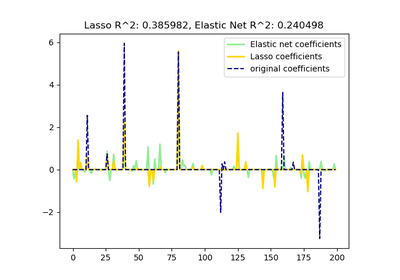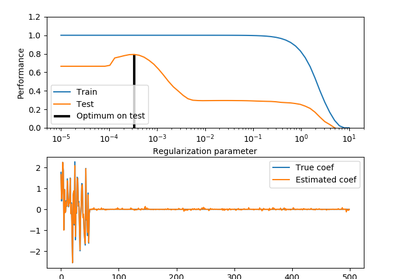# `sklearn.linear_model`.ElasticNet¶

class `sklearn.linear_model.``ElasticNet`(alpha=1.0, l1_ratio=0.5, fit_intercept=True, normalize=False, precompute=False, max_iter=1000, copy_X=True, tol=0.0001, warm_start=False, positive=False, random_state=None, selection=’cyclic’)[source]

Linear regression with combined L1 and L2 priors as regularizer.

Minimizes the objective function:

```1 / (2 * n_samples) * ||y - Xw||^2_2
+ alpha * l1_ratio * ||w||_1
+ 0.5 * alpha * (1 - l1_ratio) * ||w||^2_2
```

If you are interested in controlling the L1 and L2 penalty separately, keep in mind that this is equivalent to:

```a * L1 + b * L2
```

where:

```alpha = a + b and l1_ratio = a / (a + b)
```

The parameter l1_ratio corresponds to alpha in the glmnet R package while alpha corresponds to the lambda parameter in glmnet. Specifically, l1_ratio = 1 is the lasso penalty. Currently, l1_ratio <= 0.01 is not reliable, unless you supply your own sequence of alpha.

Read more in the User Guide.

Parameters: alpha : float, optional Constant that multiplies the penalty terms. Defaults to 1.0. See the notes for the exact mathematical meaning of this parameter.``alpha = 0`` is equivalent to an ordinary least square, solved by the `LinearRegression` object. For numerical reasons, using `alpha = 0` with the `Lasso` object is not advised. Given this, you should use the `LinearRegression` object. l1_ratio : float The ElasticNet mixing parameter, with `0 <= l1_ratio <= 1`. For `l1_ratio = 0` the penalty is an L2 penalty. `For l1_ratio = 1` it is an L1 penalty. For `0 < l1_ratio < 1`, the penalty is a combination of L1 and L2. fit_intercept : bool Whether the intercept should be estimated or not. If `False`, the data is assumed to be already centered. normalize : boolean, optional, default False This parameter is ignored when `fit_intercept` is set to False. If True, the regressors X will be normalized before regression by subtracting the mean and dividing by the l2-norm. If you wish to standardize, please use `sklearn.preprocessing.StandardScaler` before calling `fit` on an estimator with `normalize=False`. precompute : True | False | array-like Whether to use a precomputed Gram matrix to speed up calculations. The Gram matrix can also be passed as argument. For sparse input this option is always `True` to preserve sparsity. max_iter : int, optional The maximum number of iterations copy_X : boolean, optional, default True If `True`, X will be copied; else, it may be overwritten. tol : float, optional The tolerance for the optimization: if the updates are smaller than `tol`, the optimization code checks the dual gap for optimality and continues until it is smaller than `tol`. warm_start : bool, optional When set to `True`, reuse the solution of the previous call to fit as initialization, otherwise, just erase the previous solution. positive : bool, optional When set to `True`, forces the coefficients to be positive. random_state : int, RandomState instance or None, optional, default None The seed of the pseudo random number generator that selects a random feature to update. If int, random_state is the seed used by the random number generator; If RandomState instance, random_state is the random number generator; If None, the random number generator is the RandomState instance used by np.random. Used when `selection` == ‘random’. selection : str, default ‘cyclic’ If set to ‘random’, a random coefficient is updated every iteration rather than looping over features sequentially by default. This (setting to ‘random’) often leads to significantly faster convergence especially when tol is higher than 1e-4. coef_ : array, shape (n_features,) | (n_targets, n_features) parameter vector (w in the cost function formula) sparse_coef_ : scipy.sparse matrix, shape (n_features, 1) | (n_targets, n_features) `sparse_coef_` is a readonly property derived from `coef_` intercept_ : float | array, shape (n_targets,) independent term in decision function. n_iter_ : array-like, shape (n_targets,) number of iterations run by the coordinate descent solver to reach the specified tolerance.

`SGDRegressor`
implements elastic net regression with incremental training.
`SGDClassifier`
implements logistic regression with elastic net penalty (`SGDClassifier(loss="log", penalty="elasticnet")`).

Notes

To avoid unnecessary memory duplication the X argument of the fit method should be directly passed as a Fortran-contiguous numpy array.

Examples

```>>> from sklearn.linear_model import ElasticNet
>>> from sklearn.datasets import make_regression
>>>
>>> X, y = make_regression(n_features=2, random_state=0)
>>> regr = ElasticNet(random_state=0)
>>> regr.fit(X, y)
ElasticNet(alpha=1.0, copy_X=True, fit_intercept=True, l1_ratio=0.5,
max_iter=1000, normalize=False, positive=False, precompute=False,
random_state=0, selection='cyclic', tol=0.0001, warm_start=False)
>>> print(regr.coef_)
[ 18.83816048  64.55968825]
>>> print(regr.intercept_)
1.45126075617
>>> print(regr.predict([[0, 0]]))
[ 1.45126076]
```

Methods

 `fit`(X, y[, check_input]) Fit model with coordinate descent. `get_params`([deep]) Get parameters for this estimator. `path`(X, y[, l1_ratio, eps, n_alphas, …]) Compute elastic net path with coordinate descent `predict`(X) Predict using the linear model `score`(X, y[, sample_weight]) Returns the coefficient of determination R^2 of the prediction. `set_params`(**params) Set the parameters of this estimator.
`__init__`(alpha=1.0, l1_ratio=0.5, fit_intercept=True, normalize=False, precompute=False, max_iter=1000, copy_X=True, tol=0.0001, warm_start=False, positive=False, random_state=None, selection=’cyclic’)[source]
`fit`(X, y, check_input=True)[source]

Fit model with coordinate descent.

Parameters: X : ndarray or scipy.sparse matrix, (n_samples, n_features) Data y : ndarray, shape (n_samples,) or (n_samples, n_targets) Target. Will be cast to X’s dtype if necessary check_input : boolean, (default=True) Allow to bypass several input checking. Don’t use this parameter unless you know what you do.

Notes

Coordinate descent is an algorithm that considers each column of data at a time hence it will automatically convert the X input as a Fortran-contiguous numpy array if necessary.

To avoid memory re-allocation it is advised to allocate the initial data in memory directly using that format.

`get_params`(deep=True)[source]

Get parameters for this estimator.

Parameters: deep : boolean, optional If True, will return the parameters for this estimator and contained subobjects that are estimators. params : mapping of string to any Parameter names mapped to their values.
static `path`(X, y, l1_ratio=0.5, eps=0.001, n_alphas=100, alphas=None, precompute=’auto’, Xy=None, copy_X=True, coef_init=None, verbose=False, return_n_iter=False, positive=False, check_input=True, **params)[source]

Compute elastic net path with coordinate descent

The elastic net optimization function varies for mono and multi-outputs.

```1 / (2 * n_samples) * ||y - Xw||^2_2
+ alpha * l1_ratio * ||w||_1
+ 0.5 * alpha * (1 - l1_ratio) * ||w||^2_2
```

```(1 / (2 * n_samples)) * ||Y - XW||^Fro_2
+ alpha * l1_ratio * ||W||_21
+ 0.5 * alpha * (1 - l1_ratio) * ||W||_Fro^2
```

Where:

```||W||_21 = \sum_i \sqrt{\sum_j w_{ij}^2}
```

i.e. the sum of norm of each row.

Read more in the User Guide.

Parameters: X : {array-like}, shape (n_samples, n_features) Training data. Pass directly as Fortran-contiguous data to avoid unnecessary memory duplication. If `y` is mono-output then `X` can be sparse. y : ndarray, shape (n_samples,) or (n_samples, n_outputs) Target values l1_ratio : float, optional float between 0 and 1 passed to elastic net (scaling between l1 and l2 penalties). `l1_ratio=1` corresponds to the Lasso eps : float Length of the path. `eps=1e-3` means that `alpha_min / alpha_max = 1e-3` n_alphas : int, optional Number of alphas along the regularization path alphas : ndarray, optional List of alphas where to compute the models. If None alphas are set automatically precompute : True | False | ‘auto’ | array-like Whether to use a precomputed Gram matrix to speed up calculations. If set to `'auto'` let us decide. The Gram matrix can also be passed as argument. Xy : array-like, optional Xy = np.dot(X.T, y) that can be precomputed. It is useful only when the Gram matrix is precomputed. copy_X : boolean, optional, default True If `True`, X will be copied; else, it may be overwritten. coef_init : array, shape (n_features, ) | None The initial values of the coefficients. verbose : bool or integer Amount of verbosity. return_n_iter : bool whether to return the number of iterations or not. positive : bool, default False If set to True, forces coefficients to be positive. (Only allowed when `y.ndim == 1`). check_input : bool, default True Skip input validation checks, including the Gram matrix when provided assuming there are handled by the caller when check_input=False. **params : kwargs keyword arguments passed to the coordinate descent solver. alphas : array, shape (n_alphas,) The alphas along the path where models are computed. coefs : array, shape (n_features, n_alphas) or (n_outputs, n_features, n_alphas) Coefficients along the path. dual_gaps : array, shape (n_alphas,) The dual gaps at the end of the optimization for each alpha. n_iters : array-like, shape (n_alphas,) The number of iterations taken by the coordinate descent optimizer to reach the specified tolerance for each alpha. (Is returned when `return_n_iter` is set to True).

Notes

For an example, see examples/linear_model/plot_lasso_coordinate_descent_path.py.

`predict`(X)[source]

Predict using the linear model

Parameters: X : {array-like, sparse matrix}, shape = (n_samples, n_features) Samples. C : array, shape = (n_samples,) Returns predicted values.
`score`(X, y, sample_weight=None)[source]

Returns the coefficient of determination R^2 of the prediction.

The coefficient R^2 is defined as (1 - u/v), where u is the residual sum of squares ((y_true - y_pred) ** 2).sum() and v is the total sum of squares ((y_true - y_true.mean()) ** 2).sum(). The best possible score is 1.0 and it can be negative (because the model can be arbitrarily worse). A constant model that always predicts the expected value of y, disregarding the input features, would get a R^2 score of 0.0.

Parameters: X : array-like, shape = (n_samples, n_features) Test samples. y : array-like, shape = (n_samples) or (n_samples, n_outputs) True values for X. sample_weight : array-like, shape = [n_samples], optional Sample weights. score : float R^2 of self.predict(X) wrt. y.
`set_params`(**params)[source]

Set the parameters of this estimator.

The method works on simple estimators as well as on nested objects (such as pipelines). The latter have parameters of the form `<component>__<parameter>` so that it’s possible to update each component of a nested object.

Returns: self :
`sparse_coef_`

sparse representation of the fitted `coef_`

## Examples using `sklearn.linear_model.ElasticNet`¶Lasso and Elastic Net for Sparse SignalsTrain error vs Test error